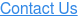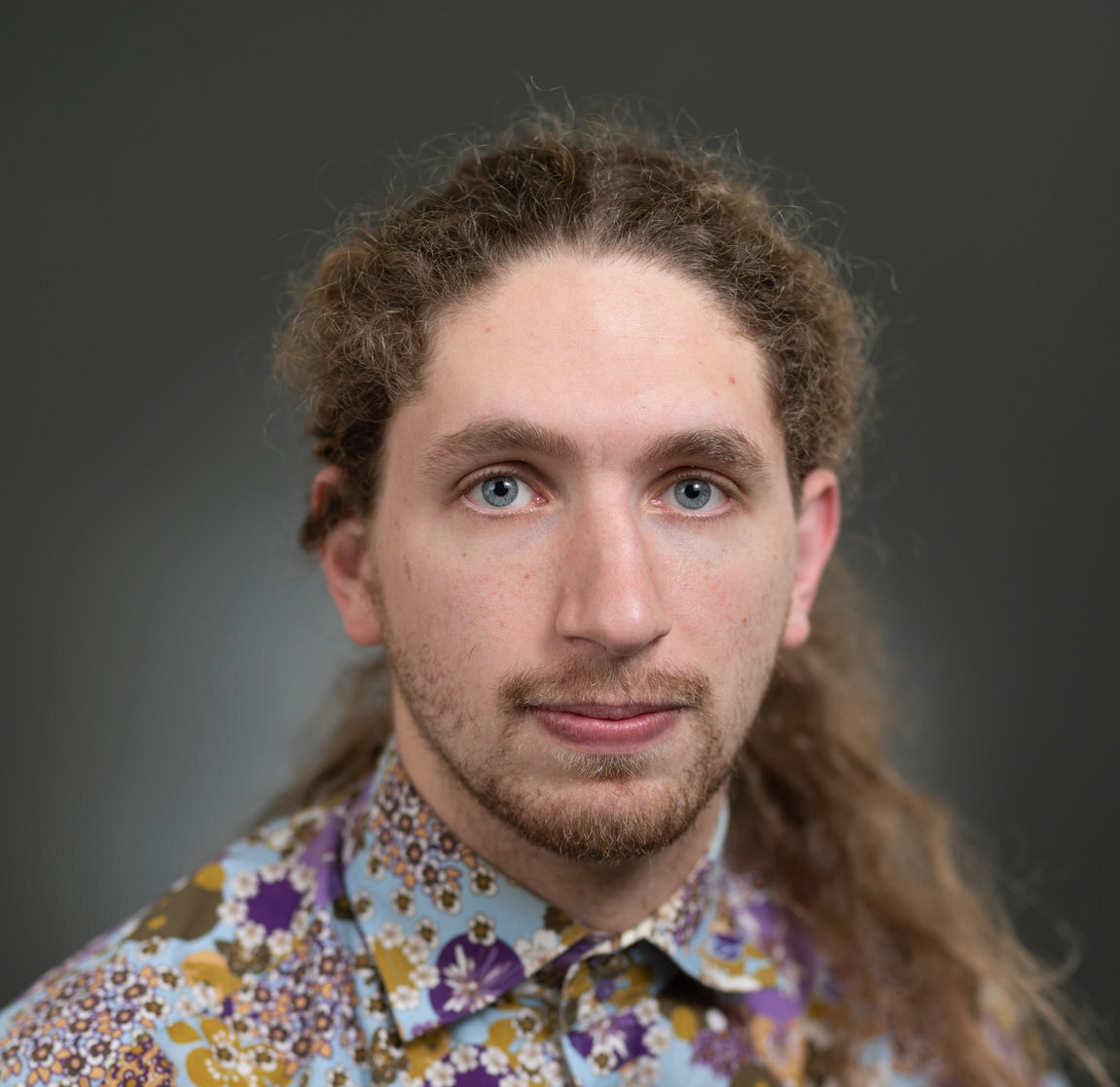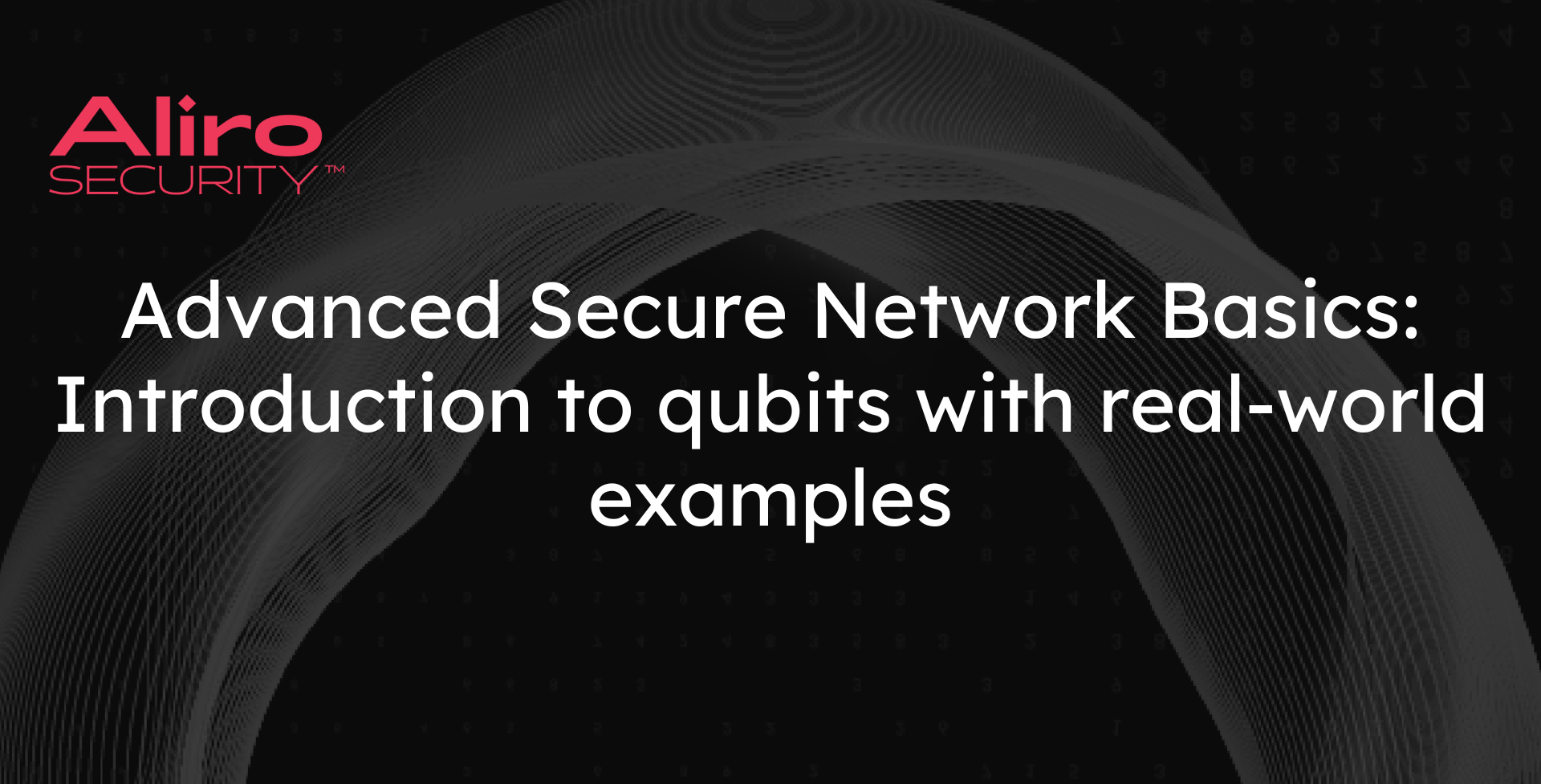# QN Basics: Introduction to qubits with real-world examplesQuantum bits—or qubits—are the fundamental building block of most quantum technologies. By harnessing the power of quantum-mechanical properties via qubits, quantum technologies can accomplish things that their classical counterparts could never match. For example, quantum technologies will help enable much more robust cybersecurity and more powerful computing. In this post, which assumes no prior knowledge of quantum technology, we will explore qubits, the quantum-mechanical properties they can display, and how they can be physically realized.

A bit—whether classical or quantum—is a single unit of information. Classical and quantum gates and circuits are “logical” functions that act on these classical and quantum bits respectively. Logical circuits are abstracted from the physical implementation of bits. This abstraction makes it possible to write classical or quantum programs that run across implementations. In this post, we will look both at the logical properties of qubits as well as how qubits are implemented in the real world, through the lens of photonic qubits.

## Computing with 0’s and 1’s: Classical bits

Classical bits are the fundamental building block of classical computers. A bit is capable of storing one piece of information. It either has value 0 or 1. A bit's value can be changed using classical gates. For example, the NOT gate sends bit value 0 to bit value 1, and bit value 1 to bit value 0. There are also gates that take in multiple bits and output a single or multiple bits of information. For example, the AND gate takes in two bits of information and outputs a single bit of information. If the two bit values inputted are 1, the AND gate outputs a 1. In all other cases, where at least one input bit is 0, the AND gate will output a 0. A circuit consists of multiple gates that the inputted bits will go through. We will stop our discussion of classical computing here, but it is amazing to realize that collections of bits, gates, and circuits are the engines that drive our computers, phones, and most other electronic devices.

## How do qubits work?

Qubits are the quantum analog to classical bits. Whereas classical bits can either have value 0 or 1, qubits can be in a combination of the $$\ket{0}$$ state and the $$\ket{1}$$ state. To capture all of the possible combination states for a qubit, we give an arbitrary qubit state $$\ket{\Psi}$$ by an equation of the form $$\ket{\Psi}=\alpha \ket{0} + \beta \ket{1}$$, where $$\alpha$$ and $$\beta$$ are complex numbers such that the sum of the squares of the magnitudes $$|\alpha|^2 + |\beta|^2$$ must equal one. There are infinitely many such pairs of $$\alpha$$ and $$\beta$$.

What do these $$\alpha$$ and $$\beta$$ values represent? They are known as amplitudes and we can use them to find the probability that the qubit will be measured in each state. Specifically, $$|\alpha|^2$$ gives the probability that when we measure $$\ket{\Psi}$$ the resulting state is $$\ket{0}$$ and $$|\beta|^2$$ gives the probability that when we measure $$\ket{\Psi}$$ the resulting state is $$\ket{1}$$. Because the sum of these values is 1, when we measure $$\ket{\Psi}$$, we must end up in either the $$\ket{0}$$ state or in the $$\ket{1}$$ state. This means that whenever we measure a qubit, even if it is in a combination of the $$\ket{0}$$ and $$\ket{1}$$ states, the qubit collapses into exactly one of these two states. We will consider a few examples of qubit states to explore the relationship between qubits and classical bits.

Consider $$\ket{\Psi}$$ when $$\alpha =1$$ and $$\beta =0$$. Since $$|\alpha|^2=1$$, there is a 100 percent chance that when we measure this qubit the resulting state is $$\ket{0}$$. Similarly, because $$|\beta|^2=0$$, there is a zero percent chance that when we measure this qubit the resulting state is $$\ket{1}$$. We can also see this by plugging these values into our equation:

$$\ket{\Psi}=1\ket{0}+0\ket{1}=\ket{0}$$

In the case where $$\alpha=0$$ and $$\beta=1$$, we would have a 100 percent chance of measuring this qubit in the $$\ket{1}$$ state. This means that the states $$\ket{0}$$ and $$\ket{1}$$ are possible qubit values. In this extreme case, our qubits look a lot like classical bits. However, things get more interesting when we look at the infinite other possible qubit states where $$\alpha$$ and $$\beta$$ are not 0.

### Superposition

When neither $$\alpha$$ nor $$\beta$$ is a 0, we say that the qubit is in a superposition of the states $$\ket{0}$$ and $$\ket{1}$$. As there are infinitely many appropriate pairs of $$\alpha$$ and $$\beta$$, this means that there are infinitely many possible different superpositions. Consider $$\ket{\Psi}$$ when $$\alpha=\frac{1}{\sqrt{2}}$$ and $$\beta=\frac{1}{\sqrt{2}}$$. Since $$|\alpha|^2$$ and $$|\beta|^2$$ both equal $$\frac{1}{2}$$, then this qubit has a 50 percent chance of being measured in the $$\ket{0}$$ state and a 50 percent chance of being measured in the $$\ket{1}$$ state. Before the measurement, we can only know the probability distribution of collapsing into the $$\ket{0}$$ state or into the $$\ket{1}$$ state, but the laws of quantum mechanics forbid us from predicting the outcome of a measurement with 100% certainty. If we independently prepared and measured two qubits in the exact same state, we could still get different states for each measurement. In the chart below, we give a few examples of states and their probability distributions for being measured in the $$\ket{0}$$ and $$\ket{1}$$ states.

 State $$\alpha$$ $$\beta$$ Probability of being measured in $$\ket{0}$$ Probability of being measured in $$\ket{1}$$ $$\ket{\Psi}=\ket{0}$$ 1 0 100% 0% $$\ket{\Psi}=\ket{1}$$ 0 1 0% 100% $$\ket{\Psi}=\frac{1}{\sqrt{2}}\ket{0}+\frac{1}{\sqrt{2}}\ket{1}$$ $$\frac{1}{\sqrt{2}}$$ $$\frac{1}{\sqrt{2}}$$ 50% 50% $$\ket{\Psi}=\frac{1}{\sqrt{2}}\ket{0}-\frac{1}{\sqrt{2}}\ket{1}$$ $$\frac{1}{\sqrt{2}}$$ $$\frac{-1}{\sqrt{2}}$$ 50% 50%

### Phase and interference

The general one-qubit state equation $$\ket{\Psi}=\alpha \ket{0}+\beta \ket{1}$$ provides more information than just the probability distributions for collapsing into the $$\ket{0}$$ state or the $$\ket{1}$$ state upon measurement. It also parameterizes the phase of the qubit. The phase of a qubit describes how the $$\ket{0}$$ and $$\ket{1}$$ states, in a superposition, interfere with one another, which will have an impact on how the qubit interacts with quantum gates.  Differences in phase can cause qubits to behave very differently even when the qubits individually have the same measurement probability distributions.

There are infinitely many different single-qubit states in equal superpositions—meaning that there is a 50% chance that they collapse into the $$\ket{0}$$ state and a 50% chance they collapse into the $$\ket{1}$$ state upon measurement. The difference between these states is their phase. For example, the two states $$\ket{\Psi_1}=\frac{1}{\sqrt{2}} \ket{0}+\frac{1}{\sqrt{2}} \ket{1}$$ and $$\ket{\Psi_2}=\frac{1}{\sqrt{2}} \ket{0}-\frac{1}{\sqrt{2}} \ket{1}$$, are both in equal superpositions—both have a 50/50 chance of ending up in either state. We might be tempted to think of these as the “same state” because of this. But, we will see an example at the end of the section of just how drastically different the resulting qubit state can be when we send these two states through the same quantum gate. These two states are actually quite useful for quantum information processing, so we give them special names: $$\ket{+}$$ and $$\ket{-}$$, respectively.

### Going beyond a single qubit

We can have multiple qubits in a system. In this section, we will take a look at two-qubit systems. A two-qubit system can be in a combination of the $$\ket{00}$$, $$\ket{01}$$, $$\ket{10}$$, and $$\ket{11}$$ states. The two numbers in these states represent the states of the two individual qubits, respectively. In the remainder of this blog, the number on the right represents the state of the first qubit and the number on the left represents the state of the second qubit. For example, for the state $$\ket{01}$$, the first qubit is in the $$\ket{1}$$ state and the second qubit is in the $$\ket{0}$$ state.

To capture all of the possible combination states for two qubits, we give an arbitrary two qubit state $$\ket{\Psi}$$ by an equation of the form $$\ket{\Psi}=\alpha_1 \ket{00} + \alpha_2 \ket{01} + \alpha_3 \ket{10} + \alpha_4 \ket{11}$$. The sum of the squared magnitudes of the four scalar values must equal 1. Again, the squared magnitude of each scalar value corresponds to the probability that when we measure $$\ket{\Psi}$$, we end up in the state the scalar is multiplying.

So, $$\ket{\Psi}$$ will be in a superposition of multiple potential outcome states if at least two of $$\alpha_1, \alpha_2, \alpha_3$$, and $$\alpha_4$$ have non-zero values. For example, one of the four Bell states, $$\ket{\Phi^+}=\frac{\ket{00}+\ket{11}}{\sqrt{2}}$$, is in a superposition of $$\ket{00}$$ and $$\ket{11}$$.

### Entanglement

The only possible resulting states when measuring $$\ket{\Phi^+}$$ are $$\ket{00}$$ and $$\ket{11}$$. This means that if we measure the state of the first qubit, then we will know what the result of measuring the second qubit must be—and vice versa. For example, if we measure the first qubit to be in the 0 state, then when the second qubit is measured it must also be in the 0 state. Entangled states are multiple-qubit superposition states in which measuring one qubit instantaneously affects the states of the other entangled qubits. Thus, $$\ket{\Phi^+}$$ is an example of an entangled state.

### Manipulating qubit systems

These quantum-mechanical properties: superposition, measurement, interference, and entanglement are precisely what allows quantum algorithms and protocols to gain advantage over their classical counterparts. We can use quantum gates to manipulate qubit systems to make use of these properties. For example, the H—or Hadamard—gate takes the state $$\ket{0}$$ to the state $$\ket{+}=\frac{\ket{0}+\ket{1}}{\sqrt{2}}$$ and the state $$\ket{1}$$ to the state $$\ket{-}=\frac{\ket{0}-\ket{1}}{\sqrt{2}}$$. Therefore, the H gate allows us to obtain a superposition from a state purely in the $$\ket{0}$$ or $$\ket{1}$$ state. Applying the H gate twice in a row cancels out its initial effect. Thus, the H gate sends the state $$\ket{+}$$ back to the state $$\ket{0}$$ and sends the state $$\ket{-}$$ back to the state $$\ket{1}$$. This demonstrates that the difference in phase between two states—even with the same measurement probability distributions—can make a large difference in how they interact with quantum gates. The H gate is often used in conjunction with another two-qubit gate called the Controlled NOT (CNOT) gate to produce entanglement and specifically the four Bell states.

We will now move away from pure theory and discuss how qubits can actually be realized as real-world systems.

## Constructing qubits in the real-world

An n-level quantum mechanical system is a property of a quantum object that has exactly n independent (physically distinguishable) possible outcome states. A qubit can be physically realized by any two-level quantum mechanical system. A qubit can also be physically realized by a multi-level (multi here meaning more than two) quantum-mechanical system, if two of these outcome states can be effectively decoupled from the rest. In a low-energy environment, these two states within the multi-level system can be used to behave exactly like a two-level system.

There are many quantum-mechanical systems that are or can behave like two-level systems, and therefore many potential candidates for what we could use to physically realize qubits. We want physical realizations of qubits and the gates that act on them to behave as similarly as possible to the theoretical qubits and gates discussed above. Noise in the system—uncontrollable errors in quantum states caused by qubits, gates, or measurements—is a major and unavoidable obstacle. To combat noise and other potential issues to the greatest extent possible, we need to choose our physical realizations carefully. There are six basic criteria of a good qubit:

1. We need to be able to isolate qubits from their surrounding environment. If qubits interfere with their environment, then they can lose the valuable information they hold.
2. Some quantum objects can still lose their information quickly even if they are isolated from the environment. So we want our qubits to have long coherence times (that is, we want our qubits to hold their information for a relatively long time).
3. We need qubits to be able to communicate effectively with other qubits. If qubits have a strong effect on one another, then it is easier to entangle them. However, we need this effect to be controllable, so that the qubits do not communicate with one another when we do not want them to do so.
4. We want qubits to be controllable and obedient so that we can carry out our quantum protocols and algorithms as intended.
5. We want physical qubit implementations that are scalable—that is, we want qubits that not only work well with near-term quantum devices, but those that are also suitable for scaling up for larger and larger quantum systems.
6. Finally, as with everything else, we want qubits to be efficiently manufacturable with minimal cost overhead.

Over the years, many different qubit implementations have been proposed and implemented. Industry leaders are split on the best path forward. In the remainder of this post, we will focus on photonic qubits, a key for quantum networks. We will assume that there is no noise present in our quantum systems when discussing photonic qubits.

### Photonic qubits

Systems of photons work well in general as representations of qubits since they satisfy—to various levels—each of the six criteria listed above. They especially shine—and are commonly used—when it comes to quantum networking. A general goal of quantum networks is to generate and distribute entanglement at a high rate with high fidelity. Photons are a natural choice for communication qubits in quantum networks because they can be transmitted over long distances with relatively little loss of information via optical fibers. There are quantum protocols, which we will discuss in future blog posts, that can even further reduce this loss.

Photons have several different two-level quantum-mechanical systems that can represent qubits, such as their horizontal and vertical polarization. We will denote horizontal and vertical polarization by $$\ket{H_p}$$ and $$\ket{V_p}$$ in the following example.

### Quantum effects right in front of your eyes: Polarized sunglasses

Believe it or not, you most likely have seen the effects of photon polarization in your own life. Linear polarization of photons plays a key role in the ways in which many daily life objects operate, such as the aptly named polarized sunglasses. Polarized sunglasses use polarization filters, also known as polarizers, to only allow for a reduced portion of the photons to pass through the filter. For example, the filter might only allow for vertically-polarized photons to pass through it. This means that the filter will measure the polarization of each photon with which it comes into contact. Any photon measured in $$\ket{V_p}$$ will pass through the filter and any photon measured in $$\ket{H_p}$$ will be absorbed by the filter. In most cases, the photons will be in a superposition of horizontal and vertical polarization. In this case, we can only know the probability that an individual photon will be measured in $$\ket{V_p}$$ or in $$\ket{H_p}$$, and therefore the probability the photon will pass through or be absorbed by the filter. A simple experiment with three polarizers is a fun and easy way to see these mechanisms in action.

### More types of photonic qubits and how to convert between them

Other examples of two-level quantum-mechanical systems of photons include:

1. circular (or helical) polarization;
2. elliptical polarization;
3. path-encoding (that is, which path a photon takes in an optical apparatus); and
4. Time-bin encoding (that is, the time interval in which a photon interacts with a specific portion of an optical apparatus).

Each of these types of photonic qubits has its own relative strengths and weaknesses. In just one example, time-bin encoded qubits—created in conjunction with classical time synchronization protocols—are robust against decoherence. However, these qubits do not communicate well with other qubits and thus are harder to entangle. It often turns out that it is easy to convert between different types of photonic qubits. Doing so, we are able to take advantage of each photonic qubit’s differing strengths and weaknesses.

We are able to convert a polarization-encoded qubit into a path-encoded qubit through the use of a polarizing beam splitter. A polarizing beam splitter will send the photons measured with vertical polarization in one direction and the photons measured with horizontal polarization in another direction. To convert between this resulting path-encoded qubit and a time-bin encoded qubit, we then need to make one of the two paths longer than the other—which we can achieve by putting a piece of glass along that path. After this, we would then re-combine the two paths into a single channel for transmission. This can be accomplished by first placing a mirror along each path so as to change that path's direction and get the two beams of light to intersect. By then placing a polarizing beam splitter at the point of intersection, the two beams of light will recombine into a single beam of light. In fact, time-bin encoded qubits are typically created by first making polarization-encoded qubits (through the use of a photon source and polarization filters) and then converting them to time-bin encoded qubits via the process we just described. One such device that can convert a polarization-encoded qubit into a time-bin encoded qubit using this methodology is called a Mach-Zehnder interferometer, which can be visualized using this awesome virtual laser lab set-up.

## Harnessing the power of qubits

To harness the powerful quantum-mechanical properties that we have discussed in this post, we need to be able to manipulate the physical realizations of qubits. To do this, each type of qubit requires its own physical realizations of the important quantum gates that can act on them. For example, a polarizing beam splitter, which we mentioned in the process mentioned above, can be used as an H gate for path-encoded photonic qubits. We mentioned at the end of the first section of this post that the H gate is often used in the process for entangling two qubits. It should be no surprise then that a polarizing beam splitter is used in several entanglement generation protocols for path-encoded photonic qubits. Entanglement is a very powerful resource for quantum technology and thus entanglement generation protocols are incredibly important. In future posts, we will look closer at how photons can be entangled whether by using beam splitters or other means.